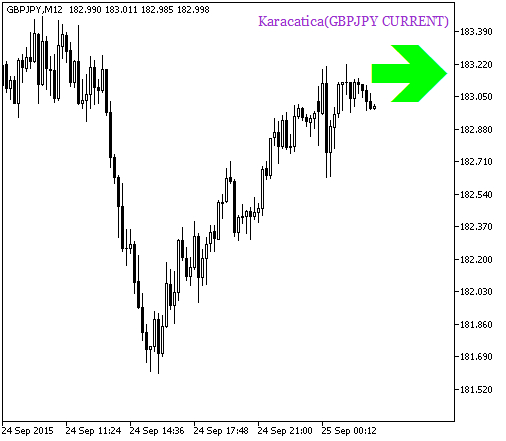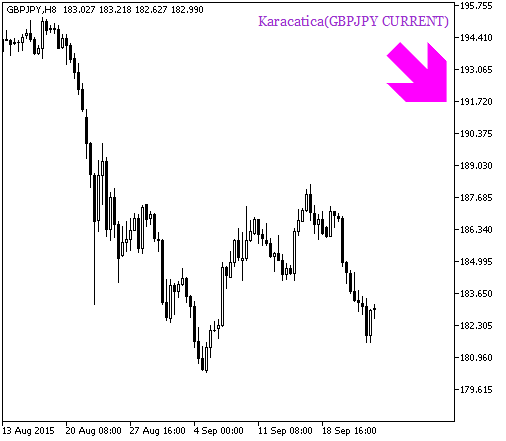# Karacatica_HTF_Signal - MetaTrader 5脚本

537

2015.11.20 10:24

2016.11.22 07:33

1. Karacatica 指标输入参数:
```//+------------------------------------------------+
//| 指标输入参数                                     |
//+------------------------------------------------+
input string Symbol_="";                               // 金融工具
input ENUM_TIMEFRAMES Timeframe=PERIOD_H6;             // 指标计算时间帧
input int iPeriod=70;                                  // 指标周期
```

2. Karacatica_HTF_Signal 指标所需要的可视化输入参数:
```//---- 指标显示设置
input uint SignalBar=0;                               // 获取信号的柱线号码 (0 - 当前柱线)
input string Symbols_Sirname=INDICATOR_NAME"_Label_"; // 指标标签名
input color UpSymol_Color=Lime;                       // 上涨符号颜色
input color DnSymol_Color=Magenta;                    // 下跌符号颜色
input color IndName_Color=DarkOrchid;                 // 指标名颜色
input uint Symbols_Size=60;                           // 信号符号大小
input uint Font_Size=10;                              // 指标名字号
input int X_1=5;                                      // 水平名位移
input int Y_1=-15;                                    // 垂直名位移
input bool ShowIndName=true;                          // 指标名显示
input ENUM_BASE_CORNER  WhatCorner=CORNER_RIGHT_UPPER;// 显示边角
input uint X_=0;                                      // 水平位移
input uint Y_=20;                                     // 垂直位移
```
3. 指标 Karacatica_HTF_Signal 所需的生成警报和声音信号的输入参数:
```//---- 提示设置
input uint AlertCount=0;                     // 提示次数
```DerivativeKalmanFilterStDevDerivative_HTFExp_derivative